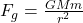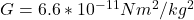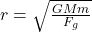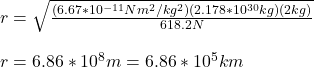## At Alpha Centauri’s surface, the gravitational force between Alpha Centauri and a 2 kg mass of hot gas has a magnitude of 618.2 N. Assuming

Question

At Alpha Centauri’s surface, the gravitational force between Alpha Centauri and a 2 kg mass of hot gas has a magnitude of 618.2 N. Assuming that Alpha Centauri is spherical, with a uniform mass of 2.178e+30 kg, what is Alpha Centauri’s mean radius?

in progress 0
7 months 2021-07-28T03:05:47+00:00 2 Answers 13 views 0

6.86 * 10^8 m

Explanation:

Parameters given:

Mass of hot gas, m = 2 kg

Gravitational Force, F = 618.2 N

Mass of Alpha Centauri, M = 2.178 * 10^30 kg

The gravitational force between two masses (the hot gas and Alpha Centauri) , m and M, at a distance, r, given as:

F = (G*M*m) / r²

Where G = gravitational constant

Therefore,

618.2 = (6.67 * 10^(-11) * 2.178 * 10^30 * 2) / r²

=> r² = (6.67 * 10^(-11) * 2.178 * 10^30 * 2) / 618.2

r² = 4.699 * 10^17 m²

=> r = 6.86 * 10^8 m

We are told that the hot gas is on the surface of Alpha Centauri, hence, the distance between both their centers is the radius of Alpha Centauri.

The mean radius of Alpha Centauri is 6.86 * 10^8 m.

The mean radius of Alpha Centauri is 6.86*10⁵km

Explanation:

Since the mass of hot gas is at Alpha Centauri’s surface, the distance between the center of mass of the star and the center of mass of the hot gas is nearly equal to the radius of Alpha Centauri. Let M be the mass of Alpha Centauri and m the mass of the hot gas. So, from the Newton’s law of universal gravitation, we have that:Where G is the gravitational constant (). Solving for the radius r, we get:Finally, plugging in the known values, we obtain:It means that the mean radius of Alpha Centauri is 6.86*10⁵km.